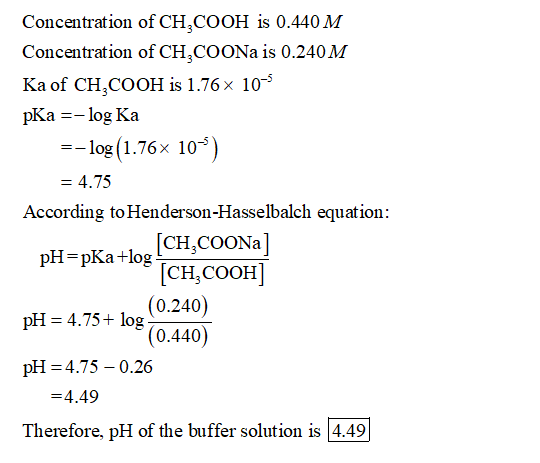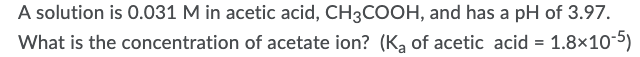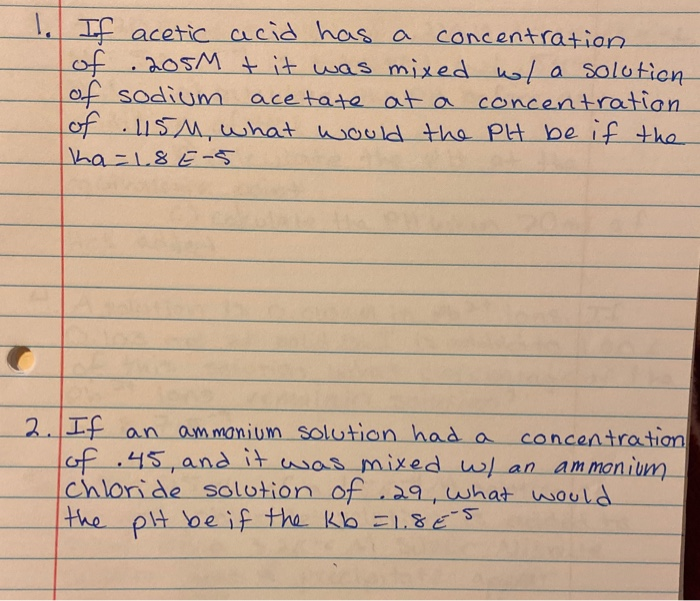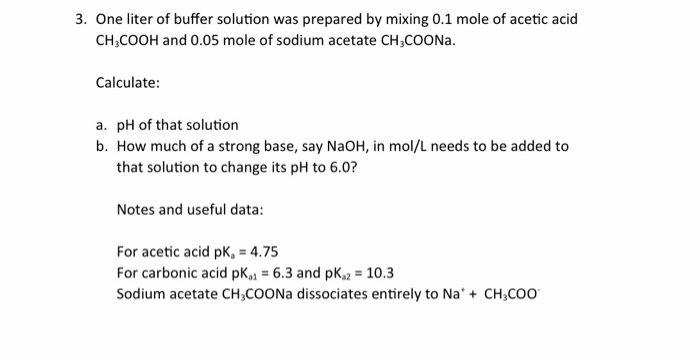Question

if 50ml of a 0.440M acetic acid solution (CH3COOH) is mixed with 50ml of a 0.240M sodium acetate solution (NaOOCCH3) what is the pH of the final solution (Ka= 1.76*10^-5)#### Earn Coins

Coins can be redeemed for fabulous gifts.

Similar Homework Help Questions
• ### calculate the ph of a buffer solution that contains 1.5 M acetic acid (CH3COOH) and 0.3...

calculate the ph of a buffer solution that contains 1.5 M acetic acid (CH3COOH) and 0.3 M sodium acetate (CH3COONa) [Ka=1.8x10-5 for acetic acid]

• ### Acetic acid (CH3COOH,CH3COOH, ?a=5.62×10−5)Ka=5.62×10−5) is a weak acid, so the salt sodium acetate (CH3COONa)CH3COONa)...

Acetic acid (CH3COOH,CH3COOH, ?a=5.62×10−5)Ka=5.62×10−5) is a weak acid, so the salt sodium acetate (CH3COONa)CH3COONa) acts as a weak base. Calculate the pH of a 0.445 M0.445 M solution of sodium acetate.

• ### what mass of sodium acetate, NaCH3COO, do we need to add to a 500 mL solution that contains 1.2g of acetic acid, CH3COO...

what mass of sodium acetate, NaCH3COO, do we need to add to a 500 mL solution that contains 1.2g of acetic acid, CH3COOH, to make a buffered solution with pH 5.0. No value change when add sodium acrtate. Ka of acid is 1.76 x10-5

• ### A solution is 0.031 M in acetic acid, CH3COOH, and has a pH of 3.97. What...A solution is 0.031 M in acetic acid, CH3COOH, and has a pH of 3.97. What is the concentration of acetate ion? (Ka of acetic acid 1.8x10-5)

• ### 1) Acetic Acid is mixed with water in an experiment. The resulting concentration of H3O+ ions...

1) Acetic Acid is mixed with water in an experiment. The resulting concentration of H3O+ ions in the reaction at equilibrium (after everything has been mixed) is 0.013M. What is the pH of the new solution at equilibrium? 2) A neutralization reaction with Acetic Acid and Sodium Hydroxide is run: CH3COOH + NaOH -> CH3COONa + H2O When the conjugate base (CH3COONa) concentration is 0.008M and the conjugate acid (CH3COOH) concentration in 0.0032M, what is the pH of the solution?...

• ### If acetic acid has a concentration of 205 t it was mixed wla solution f sodium...If acetic acid has a concentration of 205 t it was mixed wla solution f sodium acetate at a concentration of . 15M, what would the PH be if the ka=18E-5 2. If an ammonium solution had a concentration of .45, and it was mixed w/ an ammonium Chloride solution of .29, what would the pH be if the kb = 1. 865

• ### a buffer solution of pH =5.30 can be prepared by dissolving acetic acid and sodium acetate...

a buffer solution of pH =5.30 can be prepared by dissolving acetic acid and sodium acetate in water. How many moles of sodium acetate must be added to 1 L of 0.25 M acetic acid to prepare the buffer? Ka(CH3COOH)=1.8 x 10^-5

• ### What is the pH of a buffer solution which contains 0.30M HC2H3O2 (acetic acid) mixed with...

What is the pH of a buffer solution which contains 0.30M HC2H3O2 (acetic acid) mixed with 1.80M NaC2H3O2 (sodium acetate)? (Use ka chart) a. 5.53 b. 4.75 c. 3.97 d. 4.52 e. 4.98

• ### A 50.0 mL solution of 0.200 M acetic acid (CH3COOH)

a) A 50.0 mL solution of 0.200 M acetic acid (CH3COOH), 50.0 mL of 0.200 M is titrated with 0.200 M NaOH. Determine the pH.of acetic acid before any NaOH is added. The Ka of CH3COOH is 1.8 x 10-5. b) Determine the pH of the solution at the equivalent point.

• ### 3. One liter of buffer solution was prepared by mixing 0.1 mole of acetic acid CH3COOH...3. One liter of buffer solution was prepared by mixing 0.1 mole of acetic acid CH3COOH and 0.05 mole of sodium acetate CH3COONa. Calculate a. pH of that solution b. How much of a strong base, say NaOH, in mol/L needs to be added to that solution to change its pH to 6.0? Notes and useful data: For acetic acid pK4.75 For carbonic acid pKa 6.3 and pKa 10.3 Sodium acetate CH3COONa dissociates entirely to Na'CH3COO